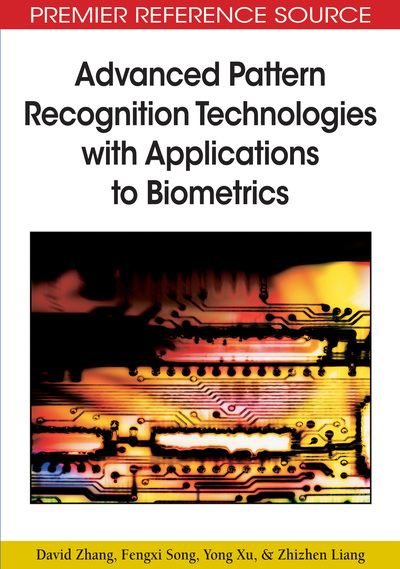# Orthogonal Discriminant Analysis Methods

David Zhang, Fengxi Song, Yong Xu, Zhizhen Liang
DOI: 10.4018/978-1-60566-200-8.ch004
OnDemand:
(Individual Chapters)
Available
\$29.50
No Current Special Offers

## Abstract

In this chapter, we first give a brief introduction to Fisher linear discriminant, Foley- Sammon discriminant, orthogonal component discriminant, and application strategies for solving the SSS problems. We then present two novel orthogonal discriminant analysis methods, orthogonalized Fisher discriminant and Fisher discriminant with Schur decomposition. At last, we compare the performance of several main orthogonal discriminant analysis methods under various SSS strategies.
Chapter Preview
Top

## Introduction

### Fisher Linear Discriminant

Fisher linear discriminant (FLD) (Duda, Hart, & Stork, 2001) operates by learning a discriminant matrix which maps a d-dimensional input space into an r-dimensional feature space by maximizing the multiple Fisher discriminant criterion.Specifically, a Fisher discriminant matrix is an optimal solution of the following optimization model:. (1)

The between-class scatter matrix SB and the within-class scatter matrix SW are defined as follows,, (2) and. (3)

It has been proved that ifis nonsingular, the matrix composed of unit eigenvectors of the matrixcorresponding to the first r largest eigenvalues is an optimal solution of the optimization model defined in Eq. (1) (Wilks, 1962). The matrixis the Fisher discriminant matrix commonly used in Fisher linear discriminant.

Since the matrixis usually asymmetric, Fisher discriminant vectors, i.e. column vectors of the Fisher discriminant matrix are unnecessary orthogonal to each other.

## Complete Chapter List

Search this Book:
Reset